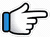# Zara Zara Behekta Hai – Keyboard Notes## Zara Zara Behekta Hai Keyboard Notes

For Zara Zara Behekta Hai Notes as Western or Indian Notations, Click –

Western – CDE FGAB

Indian – srg mpdn

Hindi – सारेग मपधनि

## Keyboard Notes for Zara Zara Behekta Hai – Indian Notation (srg mpdn)

CDE FGAB

सारेग मपधनि

### Part 1

R*r*~    R*r*~
Zara      zara

R*R*r*g*R*r*~
behekta_   hai,

R*R*r*g*R*r*~
mehekta _ hai

R*r*g*M*     r*~ R*
Aaj_ to~      mera
Or
R*R*g*M*    r*~ R*

r*~    R*r*~

R*    R*r*g*R*     r*~
main_ pyaasi_    hoon

R*R*r* g*R*        r*~
Mujhe_    bhar_  le

n~ r*     R*~ n~      d~~
apni      baahon      mein
Or
n~ r*    R*~ nR*d~~

### Repeat Part 1

R*r*~ R*r*~, R*R*r*g*R*r*~,
Zara zara,    behekta hai,

R*R*r*g*R*r*~
mehekta  hai

R*r*g*M* r*~ R* r*~ R*r*~

R* R*r*g*R* r*~,     R*R*r* g*R* r*~
main pyaasi hoon, Mujhe bhar le

n~ r* R*~ n~ d~~
apni baahon mein

### Part 2

n~   n~ r*     R*d
Hai   meri    kasam

ddR*     np~ ~
tujhko    sanam,
Or
d~ R*    np~ ~

p~ n  dM~~
door_ kahin
Or
p~ d   pM~~

R*~  n~
na     jaa

n~     r*R*d~
Yeh   doori

d~ R*n    p~~
kehti_      hai
Or
ddR*n    p~~

p~ n      dM
paas     mere
Or
p~ d     pM~~

R*R*   n~
aaja    re

### Music

Play in Flute Tone

Music part 1

M*   M*   M*~
M*p*g*~~
r* R* R*~
r*g*
r*R*n (Or R*r*n)

Music part 2

n   M*   M*   M*~
M*p*g*~~
r* R* R*~
r*g*
r*R*n (Or R*r*n)

Music part 3

n  r  r  r  r
g  g  g  g

M*  M*  M*  d*  n*  R**

Music part 4

R** n*  n*
d*  p*  M*~~

M*g*   g*M*g*p*
M* r*R*~

### Part 3

n~ r*     g*M*~     g*M*~
Yunhi    baras      baras

g*M*~    g*M*~    r*R*
kaali       ghata    barse

g*      g*~ M* r*~ r*    R*r*n
Hum   yaa__r__ bhee_g_  jaaye

g*    g*~  g*g*   g*
is     chaahat      ki

R*R*g#*M* p*M*
baarish__        mein
Or
R*g#*M*p*  g*M*
R*r*g#*M*  g*M*

### Part 4

Notes are similar to Part 3

nr*     g*M*~   g*M*~
Meri    khuli     khuli

g*M*~    g*   M*g*r*~ R*
laton       ko    suljhaaye

g     g*~ M*   r*~ r* R* r*n
Tu   apni       ungliyon   se

g*       g*  g*       g*
main   to   hoon   isi

R*R*g#*M* p*M*
khwaahish_ mein
Or
R*g#*M*p*  g*M*
R*r*g#*M*  g*M*

### Part 5

MnnM M    MR*R*M M
Sardi_ki     raaton_ mein

M~     r*~ r*   g* r*
hum    soye     rahe

R*~     n~  d~      n~
Or
R*n     d~d~      n~
R*R*  n~  d~      n~

Mn    nMM    Md dg   g
Hum   dono       tan_ha    ho,

g~  p~~   M~    gr
na   koi    bhi    rahe
Or
g    p~ pM   gr

R    r         R
is      ghar    mein

### Repeat Part 1

R*r*~ R*r*~, R*R*r*g*R*r*~,
Zara zara,     behekta hai,

R*R*r*g*R*r*~
mehekta  hai

R*r*g*M* r*~ R* r*~ R*r*~

R* R*r*g*R* r*~,  R*R*r* g*R* r*~
main pyaasi hoon,         Mujhe bhar le

n~ r* R*~ n~ d~~
apni baahon mein

Will be Updated

Ek baar ae deewane
jhootha hi sahi pyaar to kar

Main bhooli nahin
haseen mulaaqaatein
Bechain karke mujhko
mujhse yun na pher nazar

Roothega na mujhse,

Tere bina mushkil hai
jeena mera mere dil mein

Song List

Bhajan List

New Song List